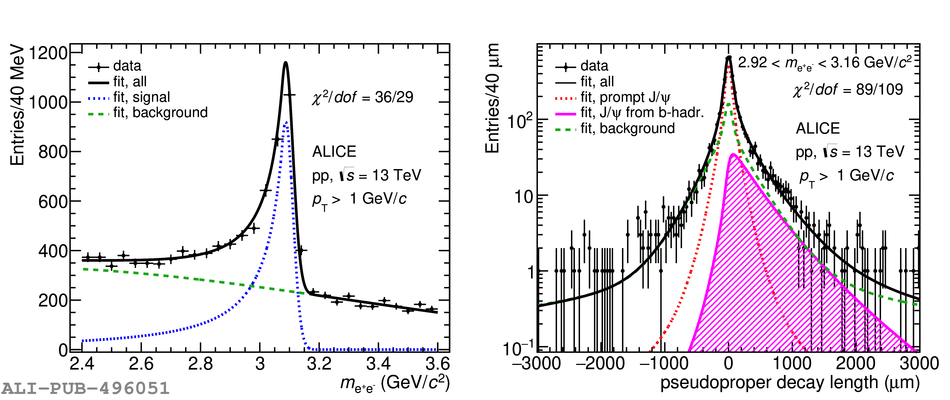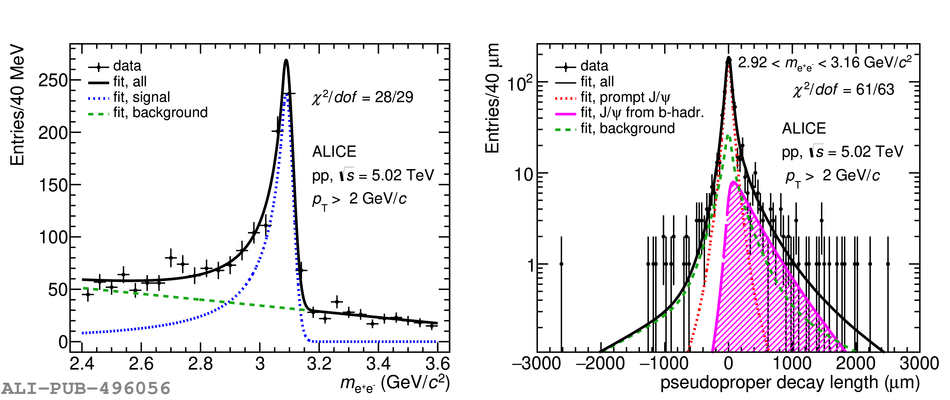# Figure 1

 Invariant mass (left panels) and pseudoproper decay length (right panels) distributions for \pJPsi candidates at midrapidity with superimposed projections of the maximum likelihood fit. Pseudoproper decay length distributions are shown for \pJPsi candidates reconstructed under the \pJPsi mass peak, i.e. for $2.92$ 1 (2) \GeVc in pp collisions at \s = 13 (5.02) TeV. The $\chi^{2}$ values, which are computed considering the binned distributions of data points and the corresponding projections of the total fit function, are also reported.